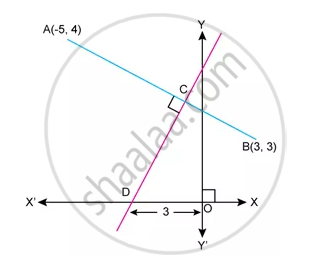Advertisement Remove all ads

# Equation of Cd - Mathematics

Sum

Find Equation of CDAdvertisement Remove all ads

#### Solution

AB and CD are Perpendicular to each other.

Thus,Product of their slops=-1

"Slope of AB" xx "Slop of CD"=-1

⇒ Slope of CD=8

Now, from graph we have coordinates of D=(-3,0)

∴ Equation of line CD is given by

y-0=8(x-3)

y=8x +24

Concept: General Equation of a Line
Is there an error in this question or solution?
Advertisement Remove all ads

#### APPEARS IN

Selina Concise Maths Class 10 ICSE
Chapter 14 Equation of a Line
Exercise 14 (E) | Q 31.2 | Page 204
Advertisement Remove all ads

#### Video TutorialsVIEW ALL 

Advertisement Remove all ads
Share
Notifications

View all notifications

Forgot password?
Course